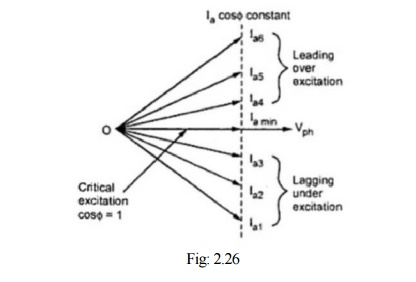Home | | Electrical Machines II | V-Curves and Inverted V-Curves

# V-Curves and Inverted V-Curves

It is clear from above discussion that if excitation is varied from very low (under excitation) to very high (over excitation) value, then current Ia decreases, becomes minimum at unity p.f. and then again increases.

V-Curves and Inverted V-Curves

It is clear from above discussion that if excitation is varied from very low (under excitation) to very high (over excitation) value, then current Ia decreases, becomes minimum at unity p.f. and then again increases. But initial lagging current becomes unity and then becomes leading in nature. This can be shown as in the Fig: 2.26.Excitation can be increased by increasing the field current passing through the field winding of synchronous motor. If graph of armature current drawn by the motor (Ia) against field current (If) is plotted, then its shape looks like an english alphabet V. If such graphs are obtained at various load conditions we get family of curves, all looking like V. Such curves are called V-curves of synchronous motor. These are shown in the Fig: 2.27 (a).

As against this, if the power factor (cos ф ) is plotted against field current (If), then the shape of the graph looks like an inverted V. Such curves obtained by plotting p.f. against If, at various load conditions are called Inverted V-curves of synchronous motor. These curves are shown in the Fig: 2.27 (b).Typically, the synchronous machine V-curves are provided by the manufacturer so that the user can determine the resulting operation under a given set of conditions.

Study Material, Lecturing Notes, Assignment, Reference, Wiki description explanation, brief detail
Electrical machines : Synchronous Motors : V-Curves and Inverted V-Curves |